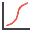The Rocscience International Conference 2021 Proceedings are now available. Read Now

# Cumulative Plot

To plot a Cumulative Distribution after a Probabilistic analysis in RocPlane:

1. Select Statistics > Plot Cumulative or the Plot Cumulativetoolbar button.
2. Select a Data Type.
3. Enter a Number of Intervals. The default is 30, but any value between 1 and 500 can be entered.
4. To display interval Markers on the plot, select the Attach Markers to Distribution check box.
5. Click OK.

The Cumulative Distribution will be generated, and the mean, standard deviation, minimum and maximum values for the data plotted, will be listed at the bottom of the Distribution.

A Cumulative Distribution is, mathematically speaking, the integral of the normalized probability density function. Practically speaking, a point on the cumulative distribution gives us the probability that a random variable will be LESS THAN OR EQUAL TO a specified value.

Simply put, if (X, Y) is a point on the cumulative distribution S-curve, then Y = the probability that the random variable will be <= X.

The data Sampler allows the user to obtain the coordinates of points on the S-curve.

The same Data Types, which can be plotted with Plot Histogram, can also be plotted as Cumulative Distributions – see the Data Type topic for details.

## Chart View Options

Several viewing options are available when generating a Cumulative plot. These options are available on the Chart menu and/or the right-click menu.